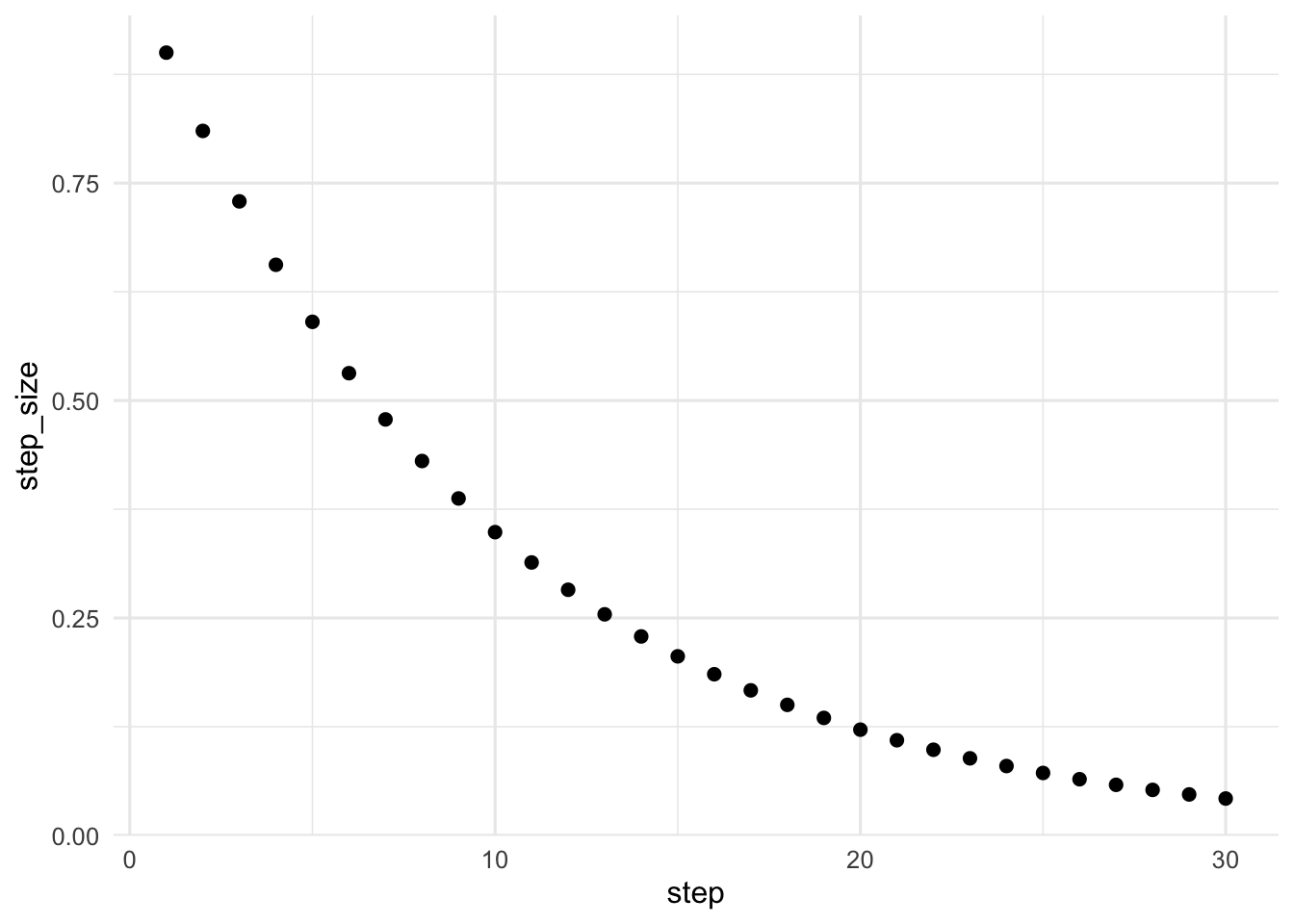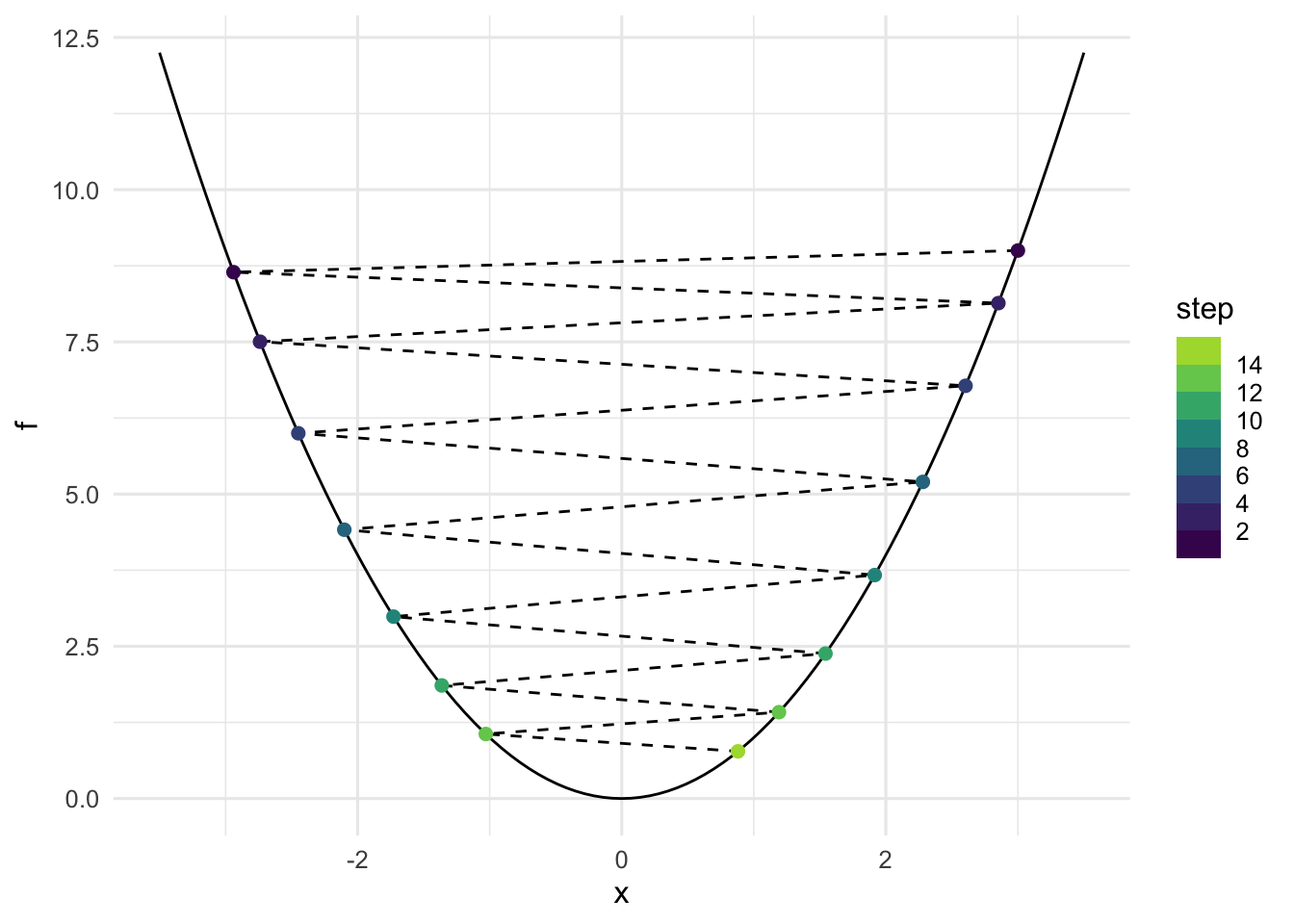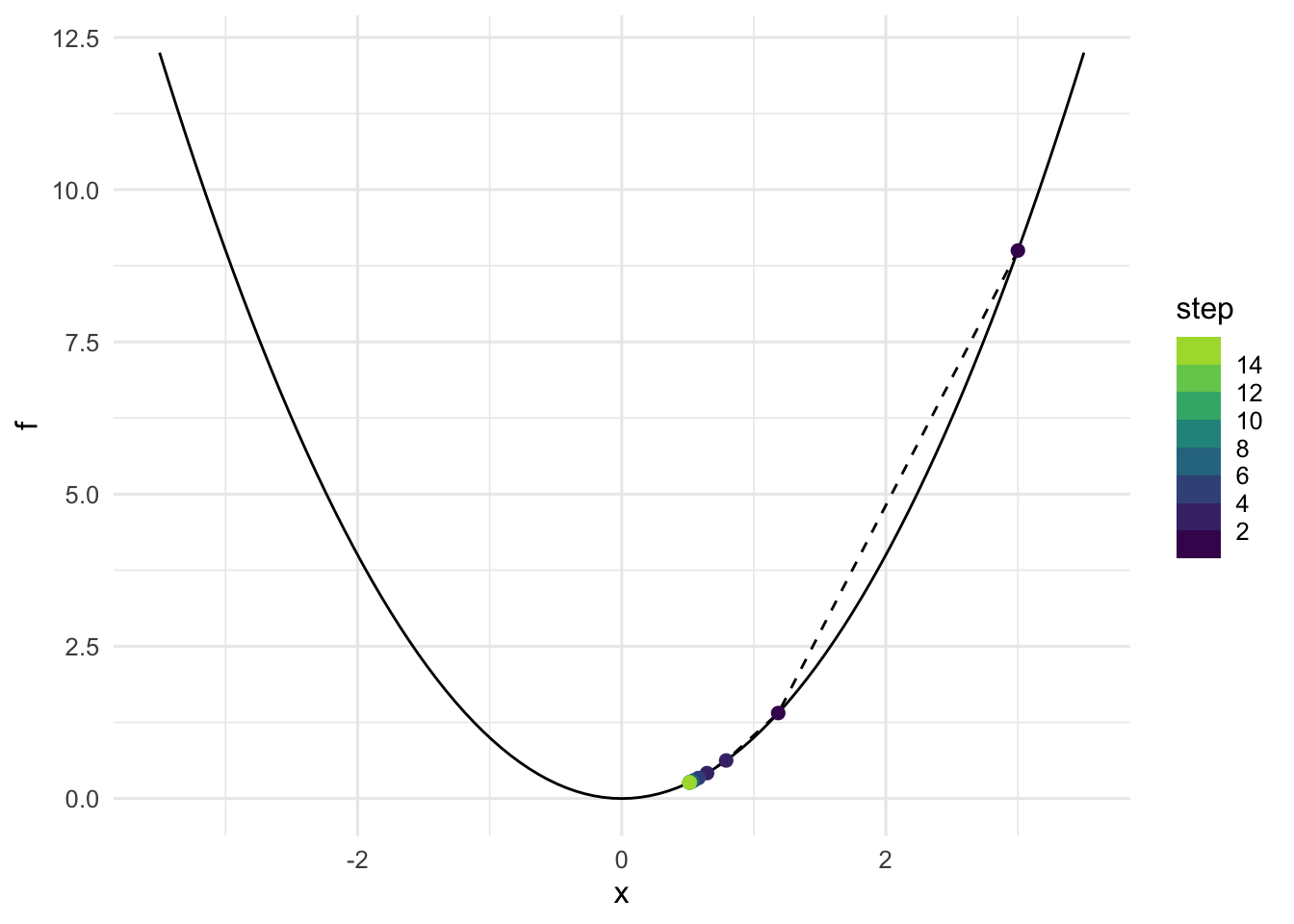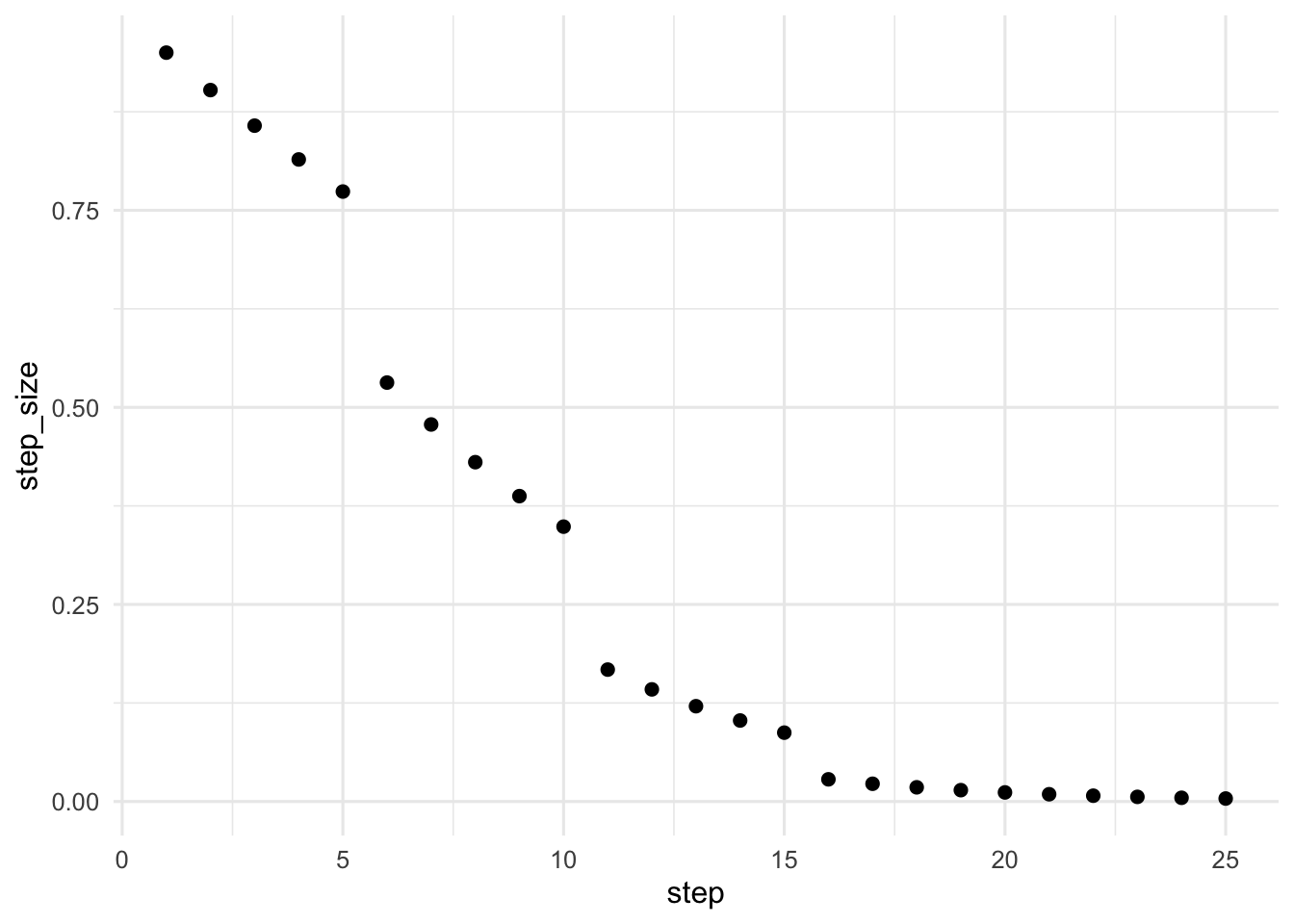## Introduction

First we introduce the basic algorithm and explore some of its important design components: step sizes and convergence checking.

The final section of this notebook shows a more sophisticated implementation of gradient descent for estimating logistic regression coefficients that uses a few advanced topics, so it’s OK to not understand all the code in a first read. Some interesting experiments to try in that section: changing the data generating parameters (n, p, sparsity, etc) or algorithm parameters (max_steps, tol).

(Note: if there’s any place where code is not displayed the source can be seen in the .Rmd file version of this notebook).

We can think of this iterative optimization algorithm as minimizing a function by taking steps in the direction of the steepest decrease (or descent). The path followed by the algorithm is like someone skiiing downhill as fast as possible by always turning in the direction with the steepest downward slope. Here’s a simple example using the function $$f(x) = x^4$$. This starts at the point $$x_0 = 1$$, and then computes a sequence of inputs $$x_1, x_2, \ldots, x_n$$ with the goal that $$f(x_n)$$ converges to a (potentially local!) minimum of $$f$$. In this case $$f$$ has a global minimum at $$x = 0$$ of $$f(0) = 0$$, and we hope our algorithm will give this correct answer.

# Function to minimize
f <- function(x) x^4
# Derivative (because it's univariate)
grad_f <- function(x) 4 * x^3
xn <- 1 # starting point
fn <- f(xn)
step_size <- .1
for (step in 1:5) {
# Update step
xn <- xn - step_size * grad_f(xn)
# Show results
cat(paste0("At step ", step,
", xn = ", round(xn, 5),
" and f(xn) = ", round(f(xn), 5)), "\n")
}
## At step 1, xn = 0.6 and f(xn) = 0.1296
## At step 2, xn = 0.5136 and f(xn) = 0.06958
## At step 3, xn = 0.45941 and f(xn) = 0.04454
## At step 4, xn = 0.42062 and f(xn) = 0.0313
## At step 5, xn = 0.39086 and f(xn) = 0.02334

Notice that xn is getting closer to 0 and f(xn) is decreasing. Perhaps if the algorithm takes more steps it will converge to the minimizer of $$f$$.

However, I’ve chosen the values here carefully. Right now it converges very slow. If we increase the number of steps from 5 to 100 it still has not yet reached even xn = 0.1, never mind the actual minimizer of 0. If we change the step_size or initial starting point xn to some other values it may converge faster, or it may diverge to infinity instead of converging to 0.

This shows that step sizes and the number of steps are important parts of any gradient descent implementation, and performance will also depend on the function (and its gradient) and starting points.

### Step sizes

The step size is a scalar multiplier of the gradient. It’s denoted $$\gamma_n$$ in the Wikipedia article and we’ll keep that convention.

We need the step size to decrease to zero so that the algorithm doesn’t get stuck jumping back and forth past the minimum value instead of getting closer to it. But how fast should it decrease?

If it starts too small or decreases too fast then gradient descent will require many more tiny steps before it reaches the optimum. On the other hand, if it decreases too slowly then gradient descent may jump around the optimum many times before the step size decreases enough for it to get closer.

#### Geometric decrease

One common approach is to take a number $$0 < \gamma < 1$$, e.g. $$\gamma \approx .9$$, and make step $$n$$ have step size $$\gamma^n$$. The step sizes then would look like this:

gamma <- .9
data.frame(step = 1:30) |>
mutate(step_size = gamma^step) |>
ggplot(aes(x = step, y = step_size)) + geom_point(size = 2)Let’s see this in action trying to minimize $$f(x) = x^2$$.

First we’ll try a value of $$\gamma$$ which is too large.

f <- function(x) x^2 # Function to optimize
max_steps <- 15
x0 <- 3 # starting point
gamma <- .995
# ... (rest of the code not displayed)

Now we plot the path of the algorithm. We can see gradient descent keeps jumping past the optimum many times, wasting computation by converging too slowly.Now we’ll try a value of $$\gamma$$ which is too small. This time gradient descent converges too fast because the step sizes become numerically close to zero even though we have not yet reached the optimum (minimum of $$f$$).

gamma <- .55Recall that at a (local) optimal value, the derivative (or gradient) should be zero (or undefined, as in the case of $$|x|$$). We can use this to check if gradient descent converged (stopped moving) because of the step size and has not yet reached an optimum:

grad_f(xnext) # numerically close to zero?
##  1.025414

The gradient isn’t close to zero, instead the algorithm has stopped because the overall step is small:

gamma^step * grad_f(xnext)
##  0.0001307192

Summary: Step sizes are decreasing too slow if gradient descent jumps around the optimum and takes a long time to converge. Step sizes are decreasing too fast if gradient descent converges but fails to reach an optimum.

#### Hand-tuning

In practice, people often try different values of $$\gamma$$, check to see if gradient descent is converging too slowly or quickly, then modify $$\gamma$$ and try again. Or they might pick a sequence of $$\gamma$$ values using multiple phases that looks something like this:Using these step sizes to minimize $$f(x) = x^2$$ again, this time the result would converge better: